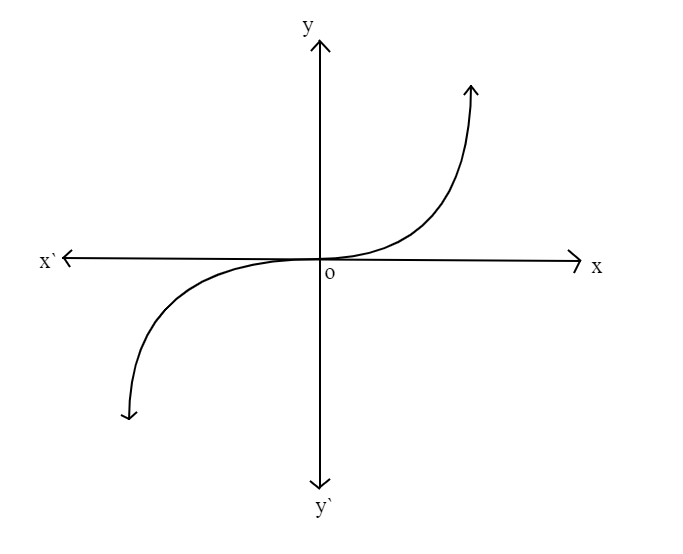Courses
Courses for Kids
Free study material
Free LIVE classes
MoreLIVE
Join Vedantu’s FREE Mastercalss

# If $f:R \to R$, then $f\left( x \right) = x\left| x \right|$ will be A. many-one-ontoB. one-one-ontoC. many-one-intoD. one-one-intoVerified
360.6k+ views
Hint: Here $R$ means a set of real numbers. The function $f:R \to R$ implies that the domain of the given function is $R$and corresponding range is also $R$. In simple words, if you put real numbers in the function you will get a set of real numbers. Plot the graph for the given function. So, use this concept to reach the solution of the problem.

Given, $f:R \to R$, $f\left( x \right) = x\left| x \right|$
This function can be redefined as
$f\left( x \right) = \left\{ {\begin{array}{*{20}{c}} { - {x^2},}&{x < 0} \\ {0,}&{x = 0} \\ {{x^2}}&{x > 0} \end{array}} \right\}$
If we plot the graph for $f\left( x \right)$, it will beHere the function $f:R \to R$ is bijective since its graph meets every horizontal and vertical line exactly once.
Since, the graph of $f\left( x \right)$ shows that it is a bijective (one-one-onto) function.
Thus, the correct option is D. one-one-into.

Note: Here a one-one function is a function of which the answers never repeat. If each element in the codomain ‘Y’ has at least one pre-image in the domain ‘X’ then the function is said to be onto. For a bijective function both one-one and onto functions must be satisfied.
Last updated date: 24th Sep 2023
Total views: 360.6k
Views today: 7.60k